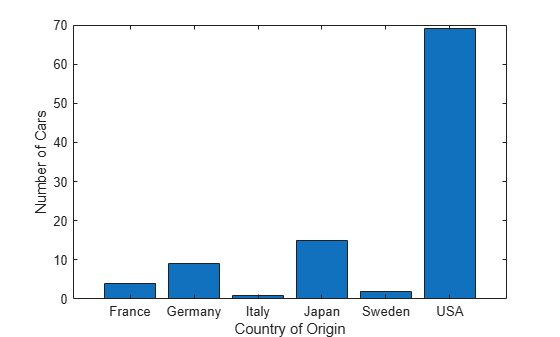tabulate

Frequency table

Description

example

tabulate(x) displays a frequency table of the data in the vector x. For each unique value in x, the tabulate function shows the number of instances and percentage of that value in x. See tbl.

example

tbl = tabulate(x) returns the frequency table tbl as a numeric matrix when x is numeric and as a cell array otherwise.

Examples

collapse all

Create a frequency table for a vector of data.

Load the patients data set. Display the first five entries of the Gender variable. Each value indicates the gender of a patient.

Gender(1:5)
ans = 5x1 cell
{'Male'  }
{'Male'  }
{'Female'}
{'Female'}
{'Female'}

Generate a frequency table that shows the number and percentage of Male and Female patients in the data set.

tabulate(Gender)
Value    Count   Percent
Male       47     47.00%
Female       53     53.00%

Create a frequency table for a vector of positive integers. By default, if a vector x contains only positive integers, then tabulate returns 0 counts for the integers between 1 and max(x) that do not appear in x. To avoid this behavior, convert the vector x to a categorical vector before calling tabulate.

Load the patients data set. Display the first five entries of the Height variable. Each value indicates the height, in inches, of a patient.

Height(1:5)
ans = 5×1

71
69
64
67
64

Create a frequency table that shows, in its second and third columns, the number and percentage of patients in the data set that have a particular height. Display the first five entries and the last five entries of the matrix that tabulate returns. tbl contains one row for each height between 1 and 72 inches, where 72 is the maximum height value in Height.

tbl = tabulate(Height);
first = tbl(1:5,:)
first = 5×3

1     0     0
2     0     0
3     0     0
4     0     0
5     0     0

last = tbl(end-4:end,:)
last = 5×3

68    15    15
69     8     8
70    11    11
71    10    10
72     4     4

Generate a frequency table that shows Count and Percent values only for heights that appear in the Height variable. Convert Height to a categorical variable, and then call the tabulate function.

newHeight = categorical(Height);
tabulate(newHeight)
Value    Count   Percent
60        1      1.00%
62        3      3.00%
63        7      7.00%
64       12     12.00%
65        8      8.00%
66       15     15.00%
67        6      6.00%
68       15     15.00%
69        8      8.00%
70       11     11.00%
71       10     10.00%
72        4      4.00%

Create a frequency table from a character array by using tabulate. Convert the resulting cell array to a table array, and visualize the results.

Load the carsmall data set. Tabulate the data in the Origin variable, which shows the country of origin of each car in the data set. Convert the resulting cell array tbl to a table array t. Change the Value column to a categorical vector.

tbl = tabulate(Origin);
t = cell2table(tbl,'VariableNames', ...
{'Value','Count','Percent'});
t.Value = categorical(t.Value)
t=6×3 table
Value     Count    Percent
_______    _____    _______

USA         69        69
France       4         4
Japan       15        15
Germany      9         9
Sweden       2         2
Italy        1         1

Create a bar graph from the frequency table.

bar(t.Value,t.Count)
xlabel('Country of Origin')
ylabel('Number of Cars')Create a frequency table from a numeric vector with NaN values.

Load the carsmall data set. The MPG variable contains the miles per gallon measurement of 100 cars. For six of the cars, the MPG value is missing (NaN).

numcars = length(MPG)
numcars = 100
nanindex = isnan(MPG);
numMissingMPG = length(MPG(nanindex))
numMissingMPG = 6

Create a frequency table using MPG. Convert the matrix output from tabulate to a table, and label the table columns.

tbl = tabulate(MPG);
t = array2table(tbl,'VariableNames', ...
{'Value','Count','Percent'})
t=37×3 table
Value    Count    Percent
_____    _____    _______

9       1      1.0638
10       2      2.1277
11       1      1.0638
13       4      4.2553
14       5      5.3191
14.5       1      1.0638
15       5      5.3191
15.5       1      1.0638
16       2      2.1277
16.5       2      2.1277
17       1      1.0638
17.5       2      2.1277
18       4      4.2553
18.5       1      1.0638
19       2      2.1277
20       2      2.1277
⋮

The frequency table displays data only for the 94 cars with numeric MPG values. tabulate calculates the percentage of each MPG value in this subset of cars, not the entire set of 100 cars.

tnumcars = sum(t.Count)
tnumcars = 94

Input Arguments

collapse all

Input data, specified as a numeric vector, logical vector, categorical vector, character array, string array, or cell array of character vectors.

• If x is a numeric vector, then tbl is a numeric matrix.

• If x is a logical vector, categorical vector, character array, string array, or cell array of character vectors, then tbl is a cell array.

Note

If the elements of x are positive integers, then the frequency table includes 0 counts for the integers between 1 and max(x) that do not appear in x. For an example, see Tabulate Positive Integer Vector.

Data Types: single | double | logical | categorical | char | string | cell

Output Arguments

collapse all

Frequency table, returned as a numeric matrix or cell array. tbl includes the following information.

ColumnDescription
1st column (Value)Unique values of x
2nd column (Count)Number of instances of each value
3rd column (Percent)Percentage of each value